# Complex numbers - Why does i^2 = -1 ?

6 posts / 0 new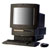Offline
Last seen: 11 years 3 weeks ago
Joined: Dec 20 2003 - 10:38
Posts: 634
Complex numbers - Why does i^2 = -1 ?

Hi All,

Ive always wondered how it is known that i squared is -1.

We know that i = -1^0.5 Using the rule (a^.5)^2 we get -1 as the answer...fine.

But if we use the rule:

Root A X Root B = Root(AB). Therefore, subtituting -1 we get Root -1 X Root -1 = Root(-1x-1)

= Root (1)
= 1.

So how do mathmaticians know that i squared = -1 not 1?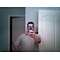Offline
Last seen: 2 days 14 hours ago
Joined: Apr 14 2004 - 17:52
Posts: 1381
Good one...

I have seen this before back in college. I cannot remember what the answer was, but it is kind of a riddle.

I will see if I can find any notes from that I may still have from that era and try to figure out what I was told at the time.

--DDTM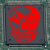Offline
Last seen: 15 years 8 months ago
Joined: Jun 8 2004 - 15:14
Posts: 102
My math teacher would love this problem

My math teacher will feel like in heaven here.He likes so much such a complex problems.

But really
If i = -1^0,5 then i = -1^1/2 which is actually Root(-1) which must be actually 1. Well ,actually ,because when I try to calculate Root of -1 in my calculator it gives me error, which is easily explainable ,because there is no number which gives negative result when being ^ .In other hands we laerned that Root of n is actually Root of |n|.This means that Root of |-n| is Root of n .But this is strange because if it was that way than my calculator woudn't give error when I try to see how much is root of -1.
Well maybe you just have to be a bit crazy if you want to be a mathematician.

Offline
Last seen: 15 years 7 months ago
Joined: Dec 26 2003 - 16:21
Posts: 584
My current(and likely permanent) knowledge of maths:

One iMac at \$185 plus another iMac at \$249.90 equals a large waste of money.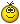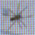Offline
Last seen: 16 years 2 days ago
Joined: Dec 20 2003 - 10:38
Posts: 71
what is x when x*x = -1?

Welcome to your first peek to the actual core of math. Its not that i = root(-1) so much as i x i = -1. In true algebra, there is no root() function, only multiplication and addition. In considering the properties of solutions to quadratic equations, mathematicians needed some way to represent that solution to the problem of x*x = -1. They chose to call that number i.

In pure math, a system is defined by a simple set of rules (called axioms) and then all of the properties of the system are derived or proven from those axioms. It is an extremely powerful way of thinking, but quickly moves away from all the familiar numerical arithmetic of early schooling. Classic algebra involves one set of rules. If you start learning more theoretical math you will soon discover that there are many algebras, each with a different set of ground rules and different strange properties.

In short i*i = -1 because mathematicians needed a symbol to represent that special something that when multiplied by itself gave the answer -1. In that sense, i is not derived so much as it was always there as soon as you conceive of an equation such as x*x = -1.Offline
Last seen: 11 years 3 weeks ago
Joined: Dec 20 2003 - 10:38
Posts: 634
Thanks everyone for the repli

Thanks everyone for the replies and helpAnd G4, thanks especially for the reply. It makes sense. I need to think about it though. Alot of new things ive never considered...